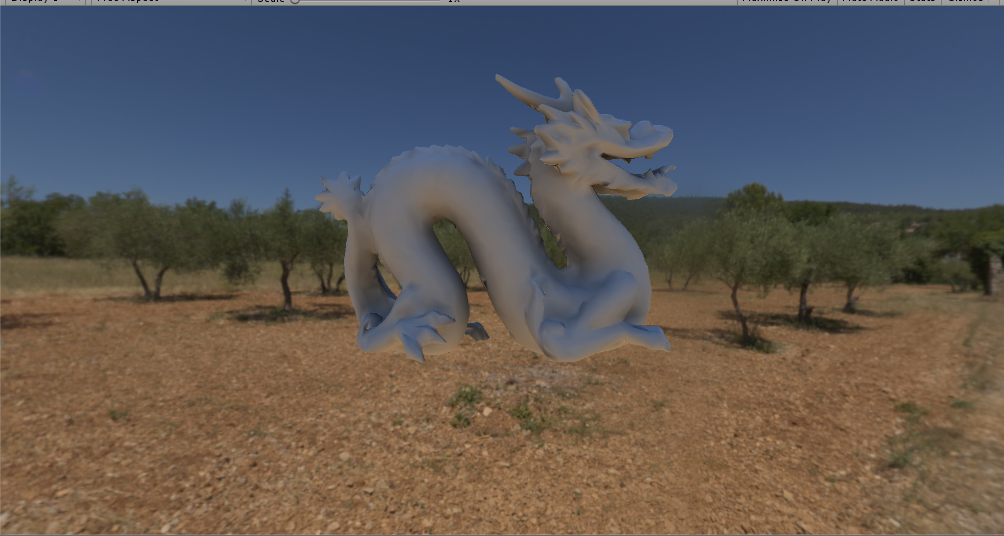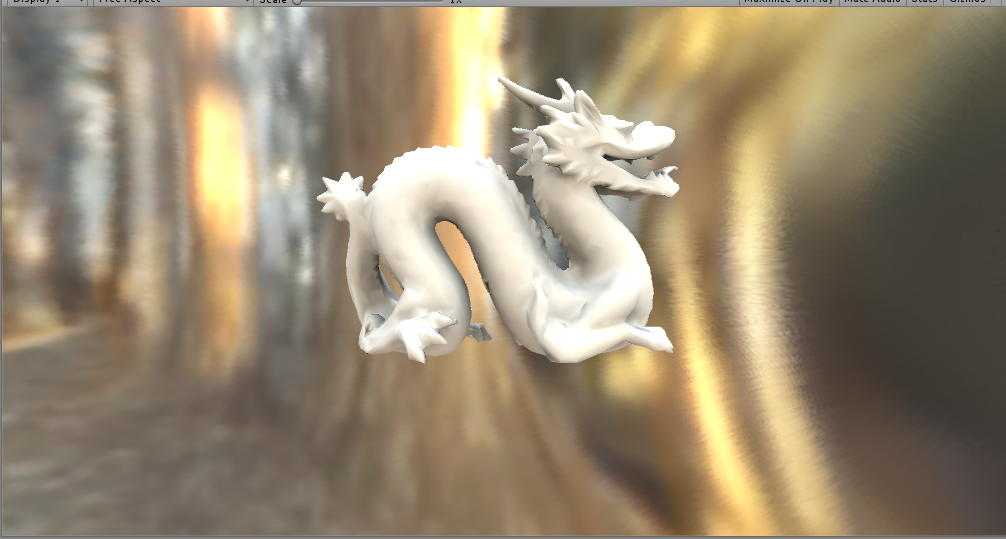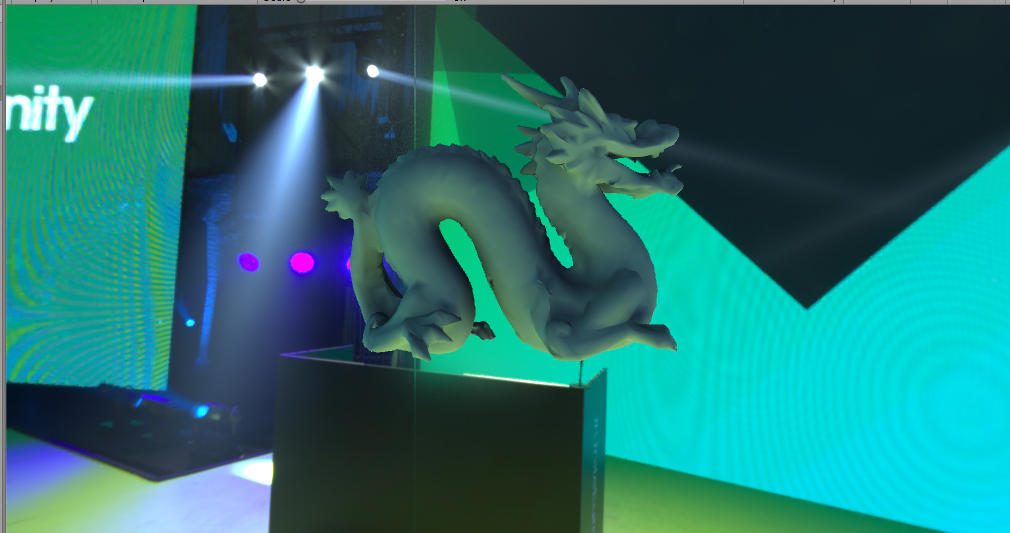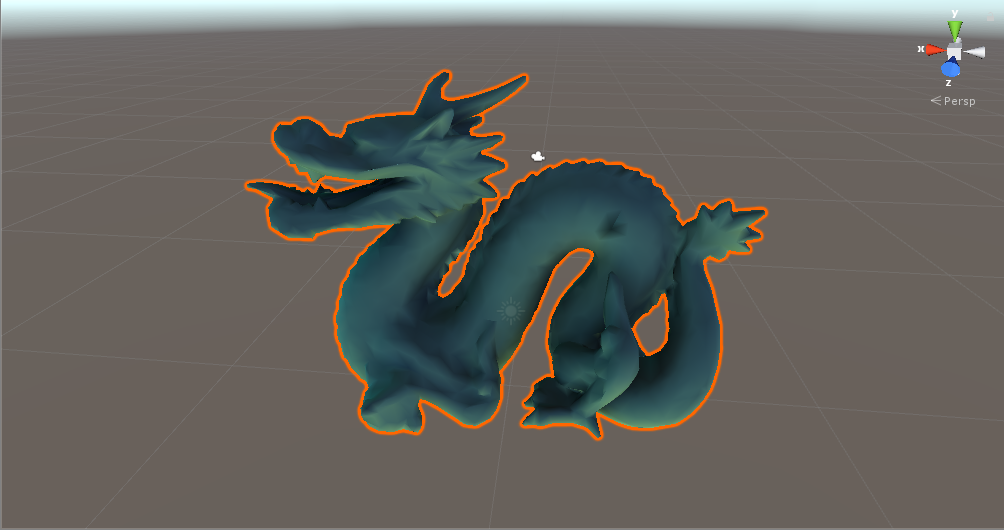# 球谐光照——简单理解和实现

1.简介：

2.解决:

2.1球谐光照与预计算：

2.1.1球谐基函数:

2.1.2积分式求解

2.1.3 内反射

2.1.4 实时绘制与旋转不变性

1.获得SH光照系数和与模型点相关的传输数据系数

2.计算实时旋转角度

3.旋转SH光照系数

4.旋转后的SH光照系数与传输数据系数相乘累加获得每点的光照颜色值。

3.实现
3.1 如何产生采样点？
private Vector2[] MCIntegrator(int maxCount)
{
Vector2[] res = new Vector2[maxCount];
for (int i = 0; i < maxCount; i++)
{
res [i].x = Random.Range (0.0f,1.0f);
res [i].y = Random.Range (0.0f,1.0f);
res [i].x = 2 * Mathf.Acos (Mathf.Sqrt(1-res[i].x));
res [i].y = 2 * res [i].y * Mathf.PI;
}
return res;
}

3.2 预计算积分的一个大致代码片段：
private void preCompute(int i)
{
Vector3 position = SHMeshObject.vertices[i];
Vector3 normal = SHMeshObject.normals[i];

Vector2[] randomVec = MCIntegrator (maxSamples);

float[] result = new float[maxPower];
for (int j = 0; j < maxPower; j++)
result [j] = 0.0f;

for(int j=0;j<maxSamples;j++)
{
Vector3 tempDir = tranfer (randomVec[j].x,randomVec[j].y);
float csn = Mathf.Clamp01(Vector3.Dot(tempDir.normalized,normal.normalized));
for (int k = 0; k < maxPower; k++)
{
int l = 0;
int m=0;
SHFunc.tranferK (k,ref l,ref m);
float y = SHFunc.SH (l,m,randomVec[j].x,randomVec[j].y);
result [k] += y * shadow * csn*albedo;
}
}

float factor = 4.0f * Mathf.PI / maxSamples;

for (int j = 0; j < maxPower; j++)
{
AllIntegratorTasks [i].coffeeSHResult [j] = result [j] * factor;
}

}

3.3SH旋转
public class PermutedMatrix
{
public PermutedMatrix(Matrix4x4 m)
{
mMat44 = m;
}

static int permute(int v)
{
if (v == 1)
return 0;
if (v == -1)
return 1;
if (v == 0)
return 2;
return 0;
}

public float GetByMN(int m,int n)
{
int row = permute (m);
int column = permute (n);

if (row == 0 && column == 0)
return mMat44.m00;
if (row == 0 && column == 1)
return mMat44.m01;
if (row == 0 && column == 2)
return mMat44.m02;

if (row == 1 && column == 0)
return mMat44.m10;
if (row == 1 && column == 1)
return mMat44.m11;
if (row == 1 && column == 2)
return mMat44.m12;

if (row == 2 && column == 0)
return mMat44.m20;
if (row == 2 && column == 1)
return mMat44.m21;
if (row == 2 && column == 2)
return mMat44.m22;

return -1;
}

private Matrix4x4 mMat44;
}

public class SHRotate
{

private static float delta(int m, int n)
{
return (m == n ? 1 : 0);
}

private static void uvw(int l,int m,int n, ref float u,ref float v,ref float w)
{
float d = delta(m, 0);
int abs_m = Mathf.Abs(m);

float denom;
if (Mathf.Abs(n) == l)
denom = (2 * l) * (2 * l - 1);

else
denom = (l + n) * (l - n);

u = Mathf.Sqrt((l + m) * (l - m) / denom);
v = 0.5f * Mathf.Sqrt((1 + d) * (l + abs_m - 1) * (l + abs_m) / denom) * (1 - 2 * d);
w = -0.5f * Mathf.Sqrt((l - abs_m - 1) * (l - abs_m) / denom) * (1 - d);
}

private static float P(int i,int l,int a,int b,PermutedMatrix R,SHRotateMatrix M)
{
if (b == -l)
{
return (R.GetByMN(i,1) * M.GetValueByBand(l - 1, a, -l + 1) + R.GetByMN(i, -1) * M.GetValueByBand(l - 1, a, l - 1));
}
else if (b == l)
{
return (R.GetByMN(i, 1) * M.GetValueByBand(l - 1, a, l - 1) - R.GetByMN(i, -1) * M.GetValueByBand(l - 1, a, -l + 1));
}
else
{
return (R.GetByMN(i, 0) * M.GetValueByBand(l - 1, a, b));
}
}

private static float U(int l,int m,int n,PermutedMatrix R,SHRotateMatrix M)
{
if (m == 0)
return (P(0, l, 0, n, R, M));

return (P(0, l, m, n, R, M));
}

private static float V(int l,int m,int n,PermutedMatrix R,SHRotateMatrix M)
{
if (m == 0)
{
float p0 = P(1, l, 1, n, R, M);
float p1 = P(-1, l, -1, n, R, M);
return (p0 + p1);
}
else if (m > 0)
{
float d = delta(m, 1);
float p0 = P(1, l, m - 1, n, R, M);
float p1 = P(-1, l, -m + 1, n, R, M);
return (p0 * Mathf.Sqrt(1 + d) - p1 * (1 - d));
}
else
{
float d = delta(m, -1);
float p0 = P(1, l, m + 1, n, R, M);
float p1 = P(-1, l, -m - 1, n, R, M);
return (p0 * (1 - d) + p1 * Mathf.Sqrt(1 - d));
}
}

private static float W(int l,int m,int n,PermutedMatrix R,SHRotateMatrix M)
{
if (m == 0)
{
return (0);
}
else if (m > 0)
{
float p0 = P(1, l, m + 1, n, R, M);
float p1 = P(-1, l, -m - 1, n, R, M);
return (p0 + p1);
}
else // m < 0
{
float p0 = P(1, l, m - 1, n, R, M);
float p1 = P(-1, l, -m + 1, n, R, M);
return (p0 - p1);
}
}

private static float M(int l,int m,int n,PermutedMatrix R,SHRotateMatrix M)
{
// First get the scalars
float u=0.0f, v=0.0f, w=0.0f;
uvw(l, m, n, ref u, ref v, ref w);

// Scale by their functions
if (u!=0.0f)
u *= U(l, m, n, R, M);
if (v!=0.0f)
v *= V(l, m, n, R, M);
if (w!=0.0f)
w *= W(l, m, n, R, M);

return (u + v + w);
}

public static Vector3[] Rotate(Vector3[] src,Matrix4x4 rot)
{
SHRotateMatrix shrm = transfer (rot,(int)Mathf.Sqrt(src.Length));
Vector3[] dest = shrm.Transform (src);
return dest;
}

public static SHRotateMatrix transfer(Matrix4x4 rot,int bands)
{
SHRotateMatrix result = new SHRotateMatrix (bands*bands);
result.SetValue (0, 0, 1);

PermutedMatrix pm = new PermutedMatrix(rot);

for (int m = -1; m <= 1; m++)
for (int n = -1; n <= 1; n++)
result.SetValueByBand (1,m,n,pm.GetByMN(m,n));

for (int band = 2; band < bands; band++)
{
for (int m = -band; m <= band; m++)
for (int n = -band; n <= band; n++)
result.SetValueByBand(band,m,n,M(band, m, n, pm,result));
}

return result;
}

}

public class SHRotateMatrix
{
public Vector3[] Transform(Vector3[] src)
{
int bands = (int)Mathf.Sqrt (mDim);
Vector3[] dest = new Vector3[src.Length];
for (int i = 0; i < dest.Length; i++)
dest [i] = Vector3.zero;
for (int l = 0; l < bands; l++)
{
for (int mo = -l; mo <= l; mo++)
{
int outputIndex = GetIndexByLM (l, mo);
Vector3 target = Vector3.zero;
for (int mi = -l; mi <= l; mi++)
{
int inputIndex = GetIndexByLM (l,mi);
float matValue = GetValueByBand (l,mo,mi);
Vector3 source = src [inputIndex];
target += source * matValue;
}

dest [outputIndex] = target;
}
}

return dest;
}

public SHRotateMatrix(int dim)
{
mDim = dim;
mMatrix = new float[mDim][];
for (int i = 0; i < mDim; i++)
{
mMatrix [i] = new float[mDim];
for (int j = 0; j < mDim; j++)
{
mMatrix [i] [j] = 0.0f;
}
}
}

public void SetValue(int i,int j,float value)
{
mMatrix [i] [j] = value;
}

public float GetValueByBand(int l,int a,int b)
{
int centre = (l + 1) * l;
return mMatrix [centre + a] [centre + b];
}

public void SetValueByBand(int l,int a,int b,float value)
{
int centre = (l + 1) * l;
mMatrix [centre + a] [centre + b] = value;
}

private int GetIndexByLM(int l,int m)
{
return (l + 1) * l + m;
}

public int mDim;
private float[][] mMatrix;
}

4.结果5.相关
https://github.com/TianYiJT/SphericalHarmonicLighting

http://www0.cs.ucl.ac.uk/staff/j.kautz/PRTCourse/

https://blog.csdn.net/baimafujinji/article/details/53869358

https://en.wikipedia.org/wiki/Spherical_harmonics

10-181万+
05-14143302-07849
04-23265
11-211万+
11-023191